# a truck travels along the horizontal circular curve of radius r=60m with a constant speed v=20m/s

a truck travels along the horizontal circular curve of radius r=60m with a constant speed v=20m/s. Determine the angular rate of rotation theta of the radial line r and the magnitude of the truck's acceleration.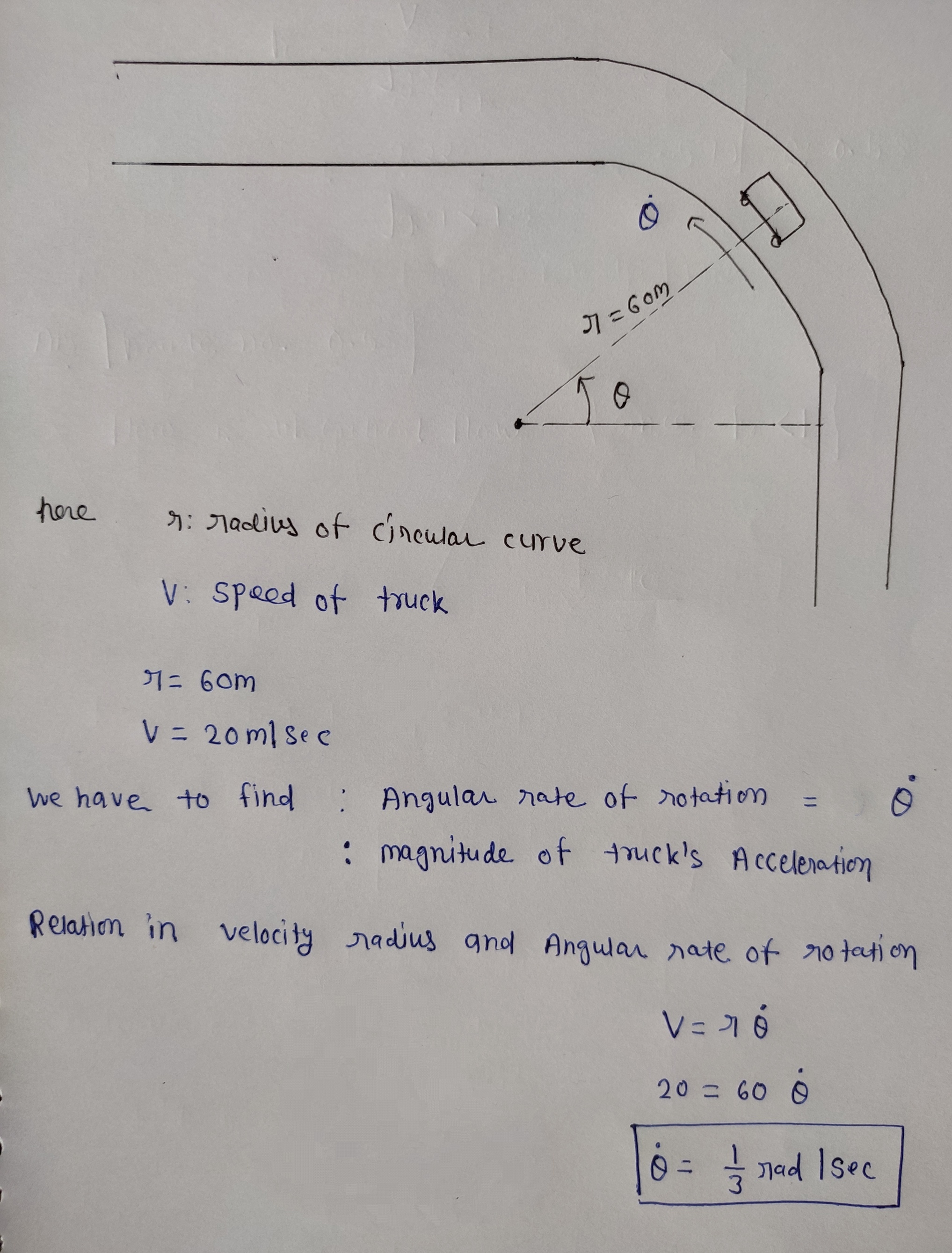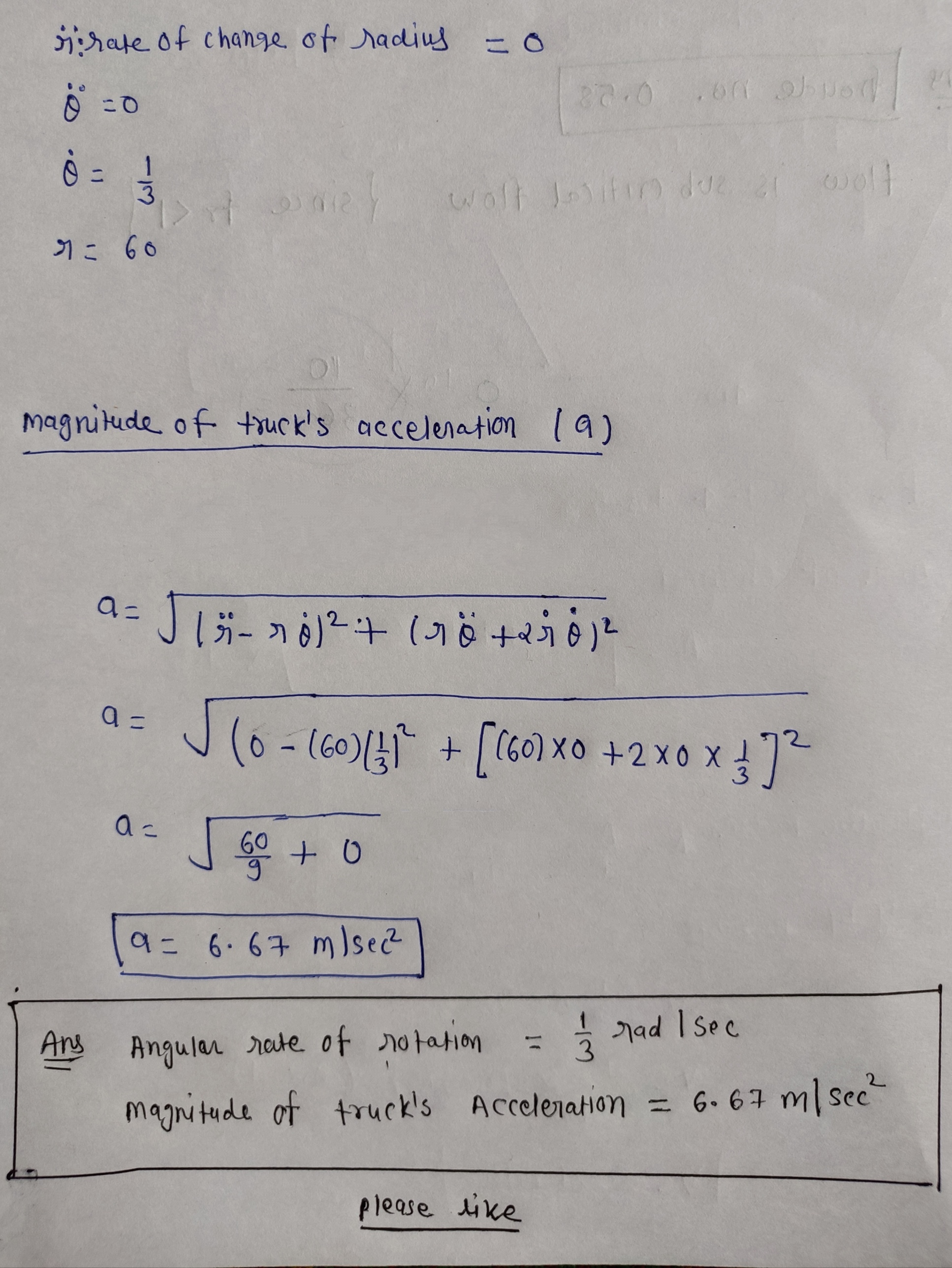The truck's acceeration is entirely centripetal, and the magnitude is
a = v^2/r

The angular rotation rate, in radians per second, is
w = v/r#### Earn Coin

Coins can be redeemed for fabulous gifts.

Similar Homework Help Questions
• ### The car travels along the circular curve of radiusr 400 ft with a constant speed of...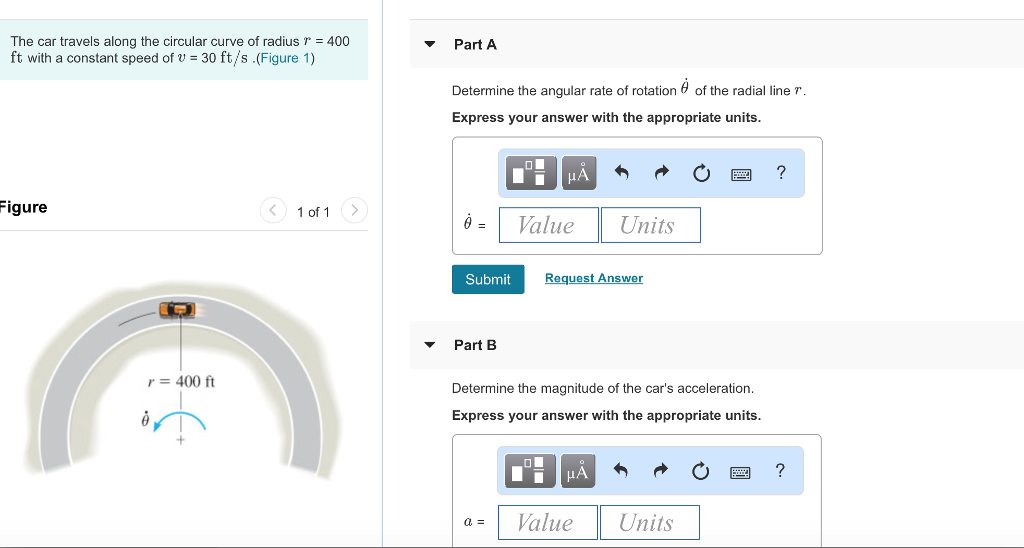The car travels along the circular curve of radiusr 400 ft with a constant speed of U = 30 ft/s .(Figure 1) ▼ Part A Determine the angular rate of rotation θ of the radial line r Express your answer with the appropriate units. Figure 1 of 1 Value Units Submit Request Answer ▼ Part B r=400 ft Determine the magnitude of the car's acceleration Express your answer with the appropriate units. a= 1 Value 1 Units

• ### Assignment 2 Problem 12.186 1 of 4 Part A truck is traveling along the horizontal circular...Assignment 2 Problem 12.186 1 of 4 Part A truck is traveling along the horizontal circular curve of radius r 60 m with a speed of 25 m/s which is increasing at 2.9 m/s?.(Figure 1 Determine the truck's radial and transverse components of acceleration Express your answers using three significant figures separated by a comma Figure 1of 1 a 10.08,2.9 m/s Subm Previous Anewers Request Answer X Incorrect; Try Again; 2 attempts remaining r 60 m Next > Provide Feedback

• ### The truck travels in a circular path having a radius of 50 m at a speed of 4 m/s. For a short...

The truck travels in a circular path having a radius of 50 m at a speed of 4 m/s. For a short distance from s = 0, its speed is increased by v = (0.05s) m/s2, where sis in meters. Determine the truck’s speed and magnitude of acceleration when it has moved s = 10 m.

• ### A particle travels counterclockwise along a circular path of radius R with a linear velocity V....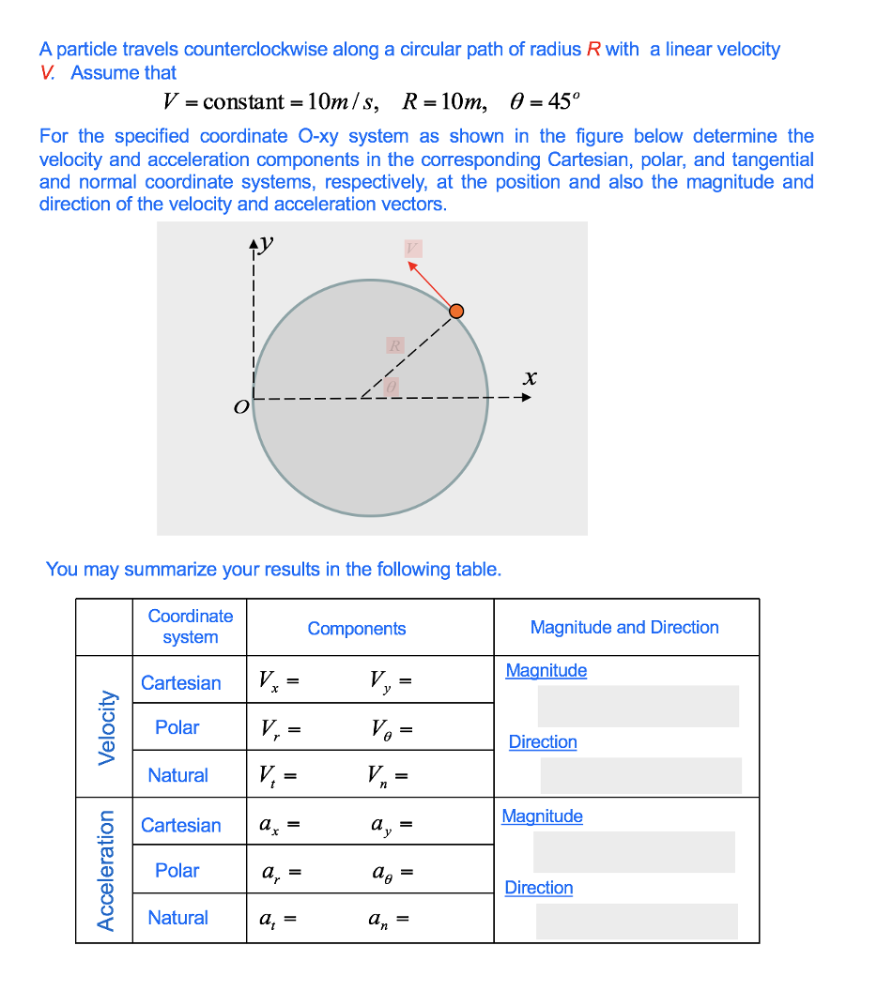A particle travels counterclockwise along a circular path of radius R with a linear velocity V. Assume that V = constant-10m /s, R-10m, θ-450 For the specified coordinate O-xy system as shown in the figure below determine the velocity and acceleration components in the corresponding Cartesian, polar, and tangential and normal coordinate systems, respectively, at the position and also the magnitude and direction of the velocity and acceleration vectors You may summarize your results in the following table. Coordinate Components...

• ### A racing car travels with a constant tangential speed of 75.0 m/s around a circular track...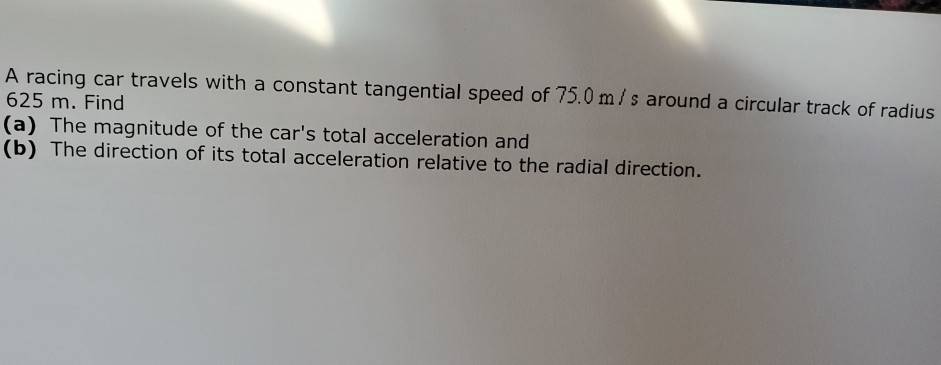A racing car travels with a constant tangential speed of 75.0 m/s around a circular track of radius 625 m. Find |(a) The magnitude of the car's total acceleration and |(b) The direction of its total acceleration relative to the radial direction.

• ### The small block P travels with constant speed v = 1.70 m/s in the circular path...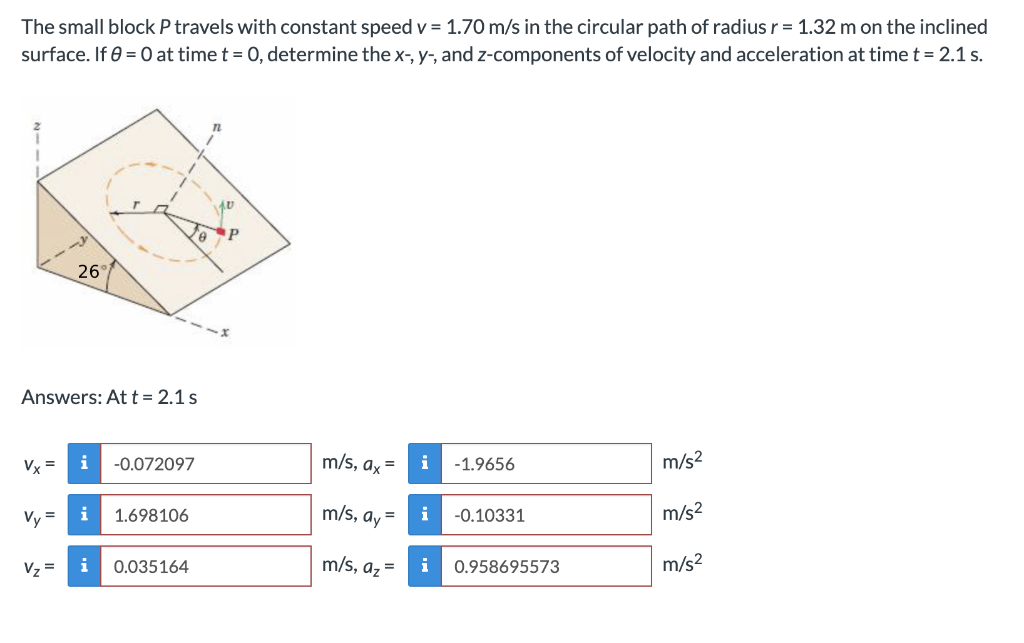The small block P travels with constant speed v = 1.70 m/s in the circular path of radius r = 1.32 m on the inclined surface. If 0 = 0 at time t = 0, determine the x-, y, and z-components of velocity and acceleration at time t = 2.1 s. Answers: At t = 2.1 s -0.072097 m/s, ax = i -1.9656 m/s2 1.698106 m/s, ay = -0.10331 m/s2 0.035164 m/s, a, = 0.958695573 m/s2 N

• ### A car travels at a constant speed around a circular track whose radius is 2.51 km....

A car travels at a constant speed around a circular track whose radius is 2.51 km. The car goes once around the track in 356 s. What is the magnitude of the centripetal acceleration of the car?

• ### 3. The point begins to move along the circular path of radius R = 30 m...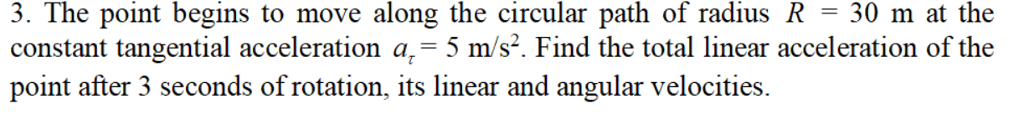3. The point begins to move along the circular path of radius R = 30 m at the constant tangential acceleration a,-5 m/s. Find the total linear acceleration of the point after 3 seconds of rotation, its linear and angular velocities.

• ### A car travels at a constant speed around a circular track whose radius is 3.0 km....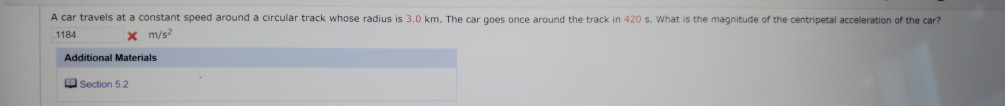A car travels at a constant speed around a circular track whose radius is 3.0 km. The car goes once around the track in 420 s. What is the magnitude of the centripetal acceleration of the car? x m/s .1184 Additional Materials Section 5.2

• ### 1. A cockroach crawls with a constant speed v'in a circular path of radius b on...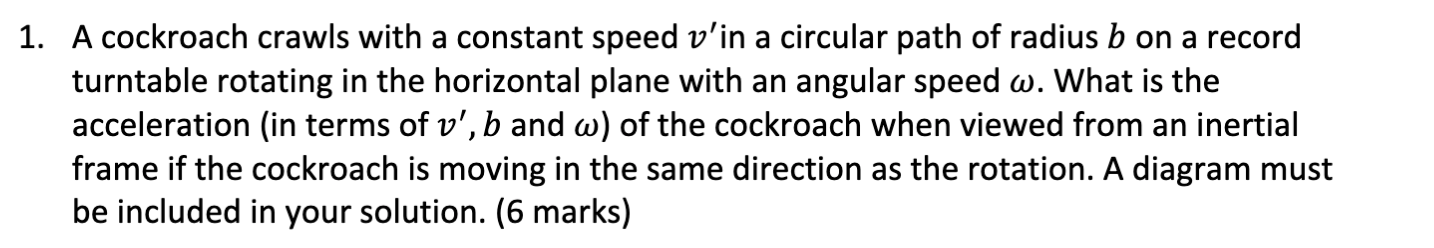1. A cockroach crawls with a constant speed v'in a circular path of radius b on a record turntable rotating in the horizontal plane with an angular speed w. What is the acceleration in terms of v', b and w) of the cockroach when viewed from an inertial frame if the cockroach is moving in the same direction as the rotation. A diagram must be included in your solution. (6 marks)